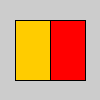Name

red()

Examplesc = color(255, 204, 0)  # Define color 'c'
fill(c)  # Use color variable 'c' as fill color
rect(15, 20, 35, 60)  # Draw left rectangle

redValue = red(c)  # Get red in 'c'
print(redValue)  # Print "255.0"
fill(redValue, 0, 0)  # Use 'redValue' in fill
rect(50, 20, 35, 60)  # Draw right rectangle
Description Extracts the red value from a color, scaled to match current colorMode(). The value is always returned as a float, so be careful not to assign it to an int value.

The red() function is easy to use and understand, but it is slower than a technique called bit shifting. When working in colorMode(RGB, 255), you can acheive the same results as red() but with greater speed by using the right shift operator (>>) with a bit mask. For example, the following two lines of code are equivalent means of getting the red value of the color value c:

r1 = red(c)  # Simpler, but slower to calculate
r2 = c >> 16 & 0xFF  # Very fast to calculate
Syntax
red(rgb)
Parameters
rgb int: any value of the color datatype
Related green()
blue()
alpha()
hue()
saturation()
brightness()
>> (right shift)

Updated on Mon Sep 21 15:53:25 2020.

If you see any errors or have comments, please let us know.